# 【图文详解】200行JS代码，带你实现代码编译器（人人都能学会）# 一、前言• React 中 JSX 转换成 JS 代码；
• 通过 Babel 将 ES6 及以上规范的代码转换成 ES5 代码；
• 通过各种 Loader 将 Less / Scss 代码转换成浏览器支持的 CSS 代码；
• 将 TypeScript 转换为 JavaScript 代码。
• and so on...

# 二、编译器介绍

## 2.1 程序运行方式

### 静态编译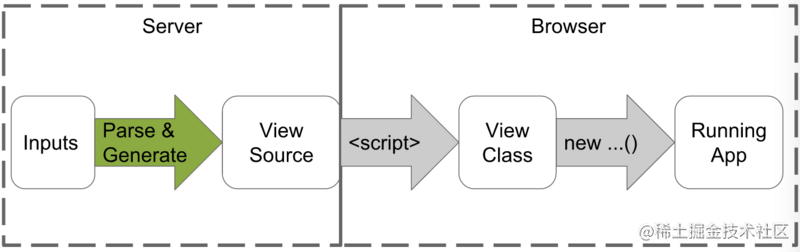（图片来自：segmentfault.com/a/119000000…

• 使用 TypeScript 开发 Angular 应用
• 运行 ngc 编译应用程序
• 使用 Angular Compiler 编译模板，一般输出 TypeScript 代码
• 运行 tsc 编译 TypeScript 代码
• 使用 Webpack 或 Gulp 等其他工具构建项目，如代码压缩、合并等
• 部署应用

### 动态解释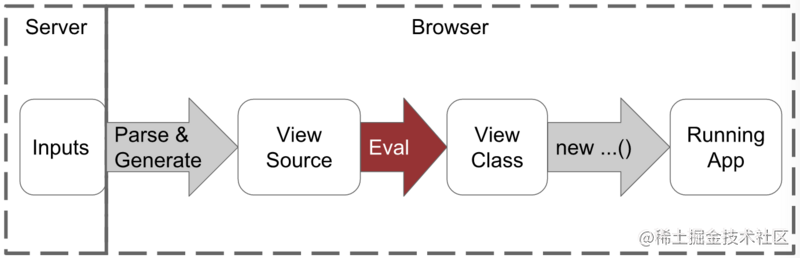（图片来自：segmentfault.com/a/119000000…

• 使用 TypeScript 开发 Angular 应用
• 运行 tsc 编译 TypeScript 代码
• 使用 Webpack 或 Gulp 等其他工具构建项目，如代码压缩、合并等
• 部署应用

### AOT vs JIT

AOT 编译流程：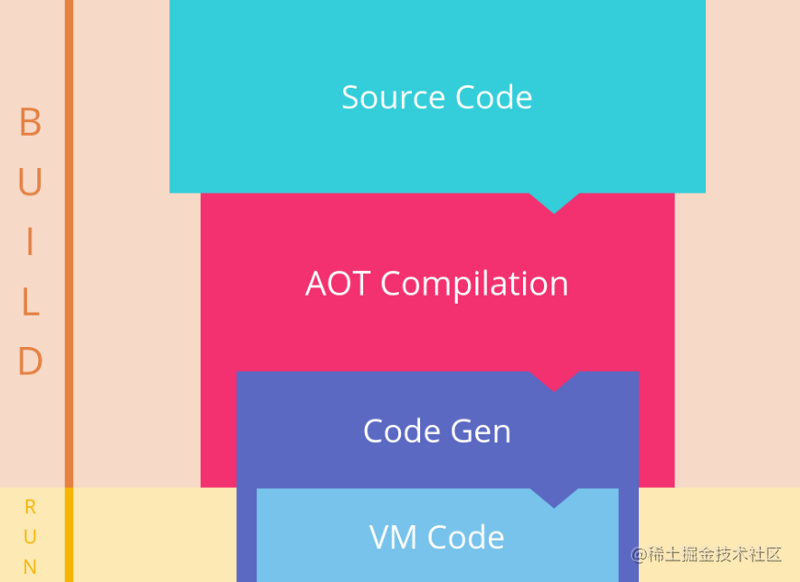（图片来自：segmentfault.com/a/119000000…

JIT 编译流程：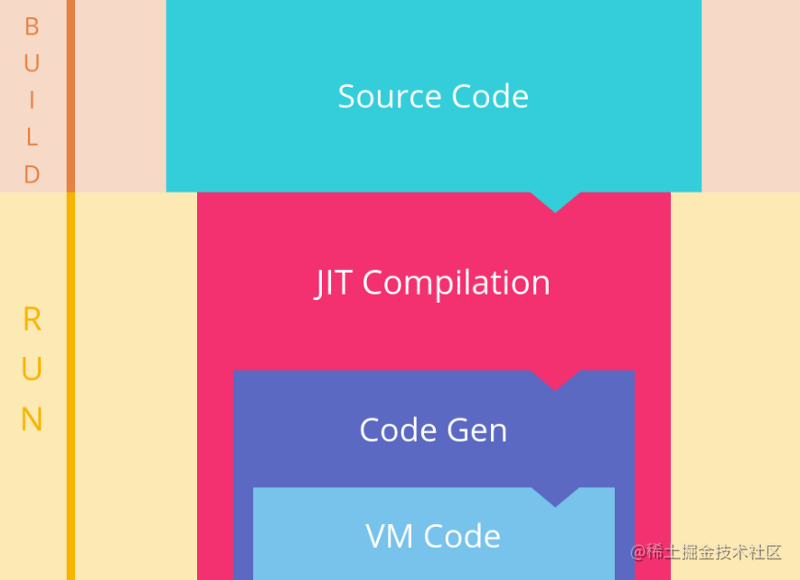（图片来自：segmentfault.com/a/119000000…

• 在客户端我们不需要导入体积庞大的 angular 编译器，这样可以减少我们 JS 脚本库的大小
• 使用 AOT 编译后的应用，不再包含任何 HTML 片段，取而代之的是编译生成的 TypeScript 代码，这样的话 TypeScript 编译器就能提前发现错误。总而言之，采用 AOT 编译模式，我们的模板是类型安全的。

## 2.2 现代编译器工作流程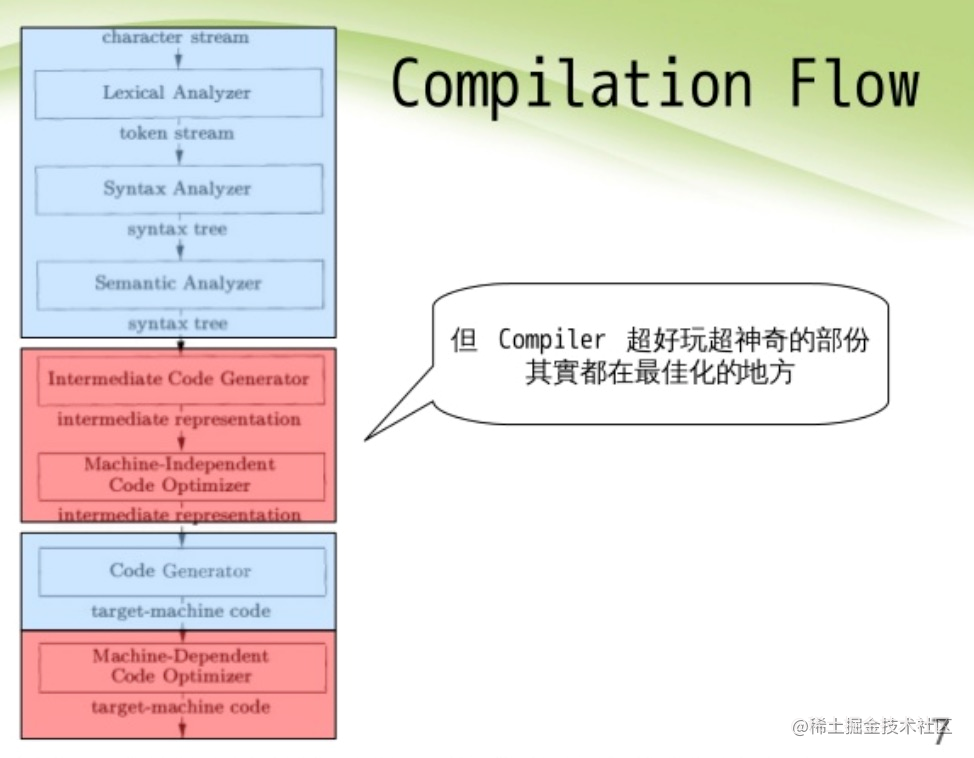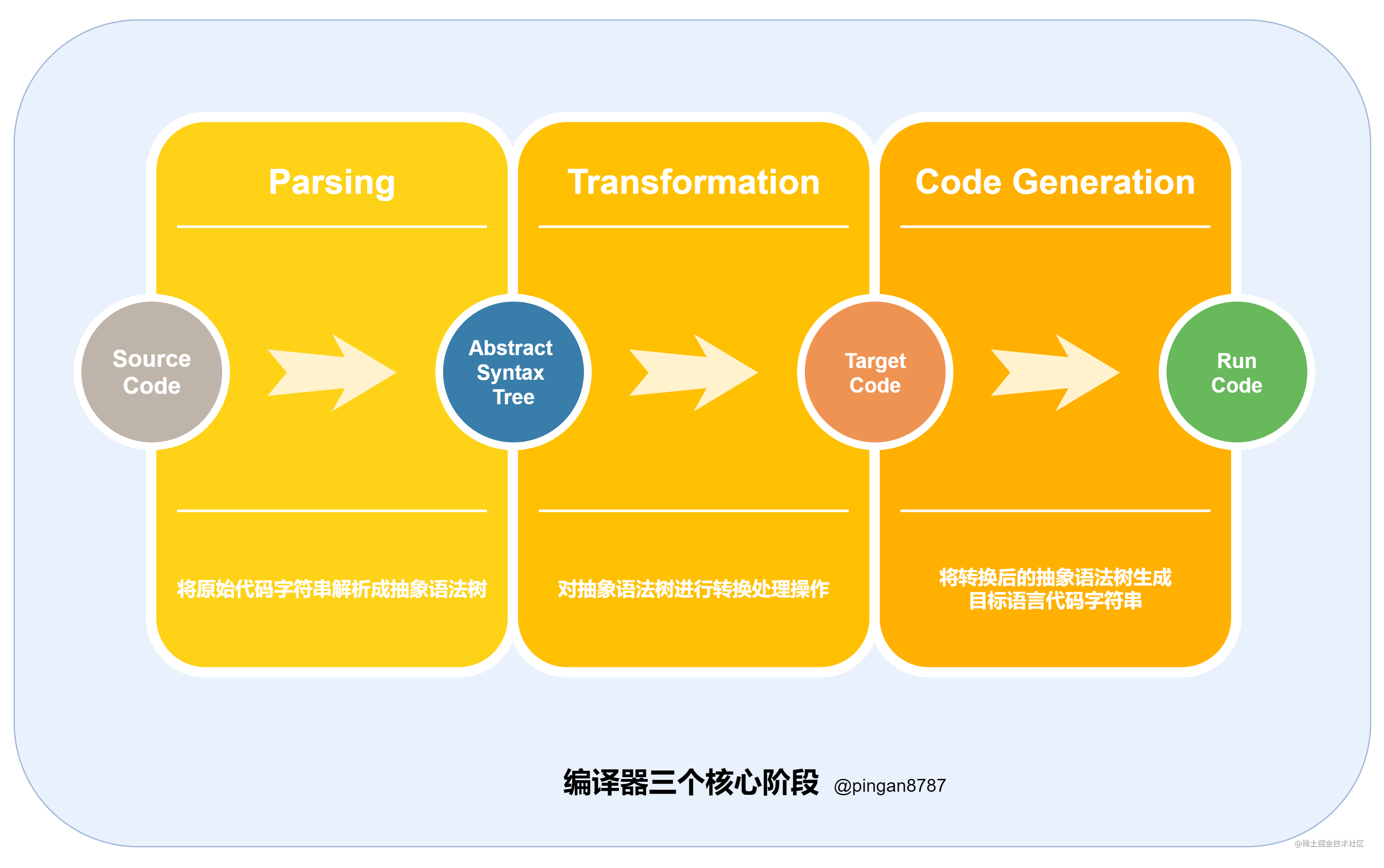1. 解析（Parsing ：通过词法分析和语法分析，将原始代码字符串解析成抽象语法树（Abstract Syntax Tree）
2. 转换（Transformation：对抽象语法树进行转换处理操作；
3. 生成代码（Code Generation：将转换之后的 AST 对象生成目标语言代码字符串。

# 三、编译器实现

Lisp 风格（编译前）JavaScript 风格（编译后）
4 - 2(subtract 4 2)subtract(4, 2)## 3.1 The Super Tiny Compiler 工作流程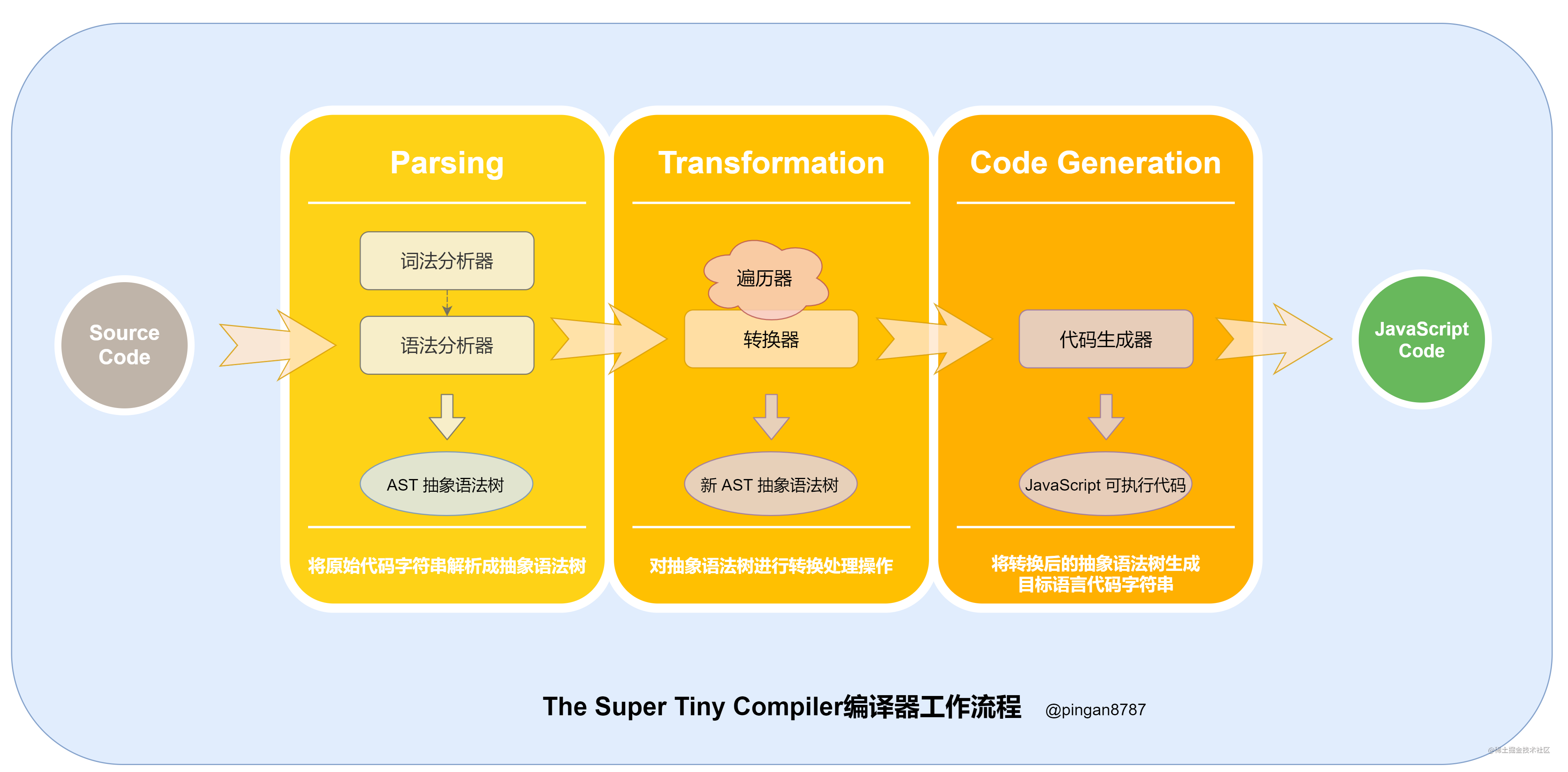1. 执行入口函数，输入原始代码字符串作为参数；
``````// 原始代码字符串

1. 进入解析阶段（Parsing），原始代码字符串通过词法分析器（Tokenizer）转换为词法单元数组，然后再通过 语法分析器（Parser）词法单元数组转换为抽象语法树（Abstract Syntax Tree 简称 AST），并返回；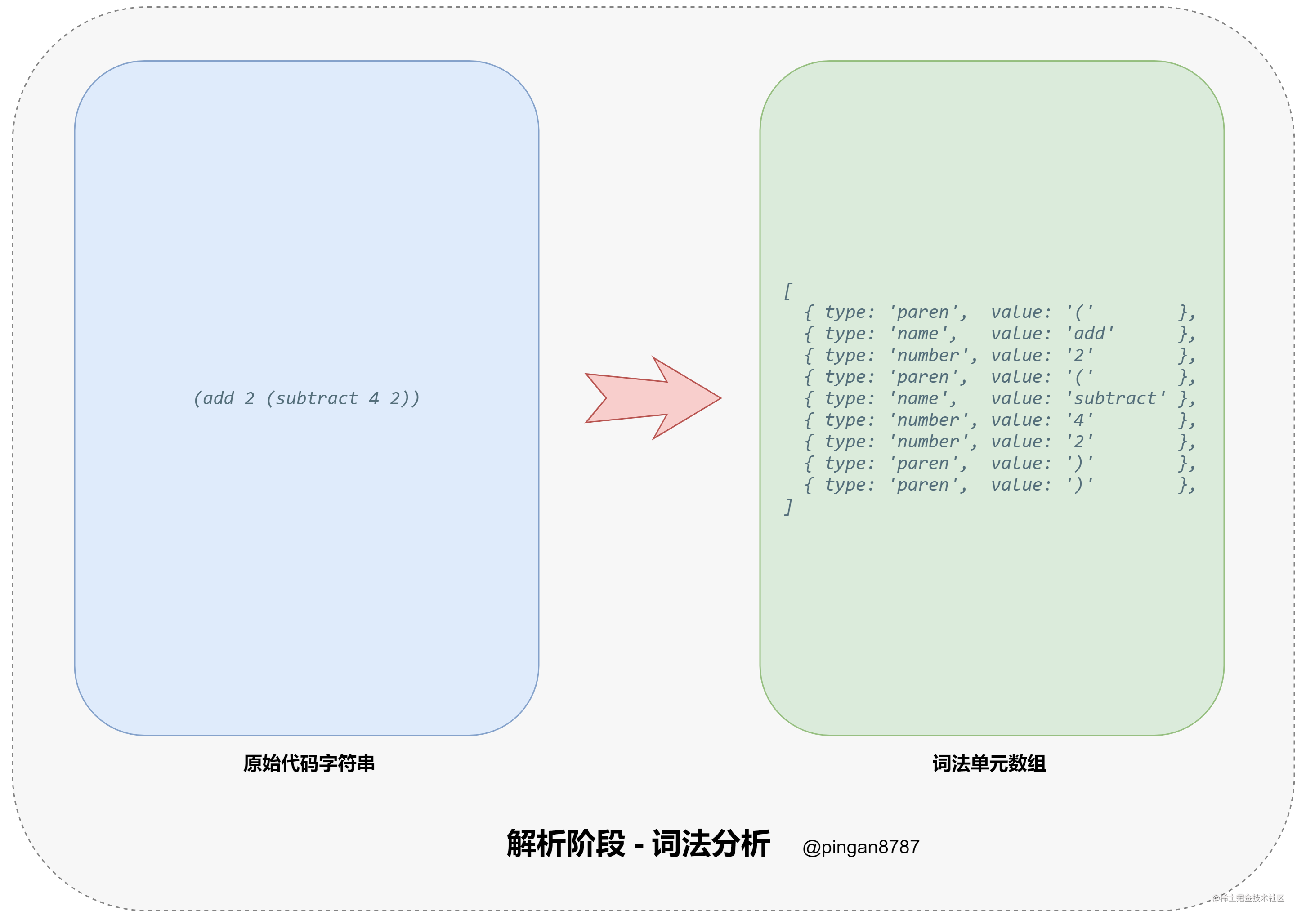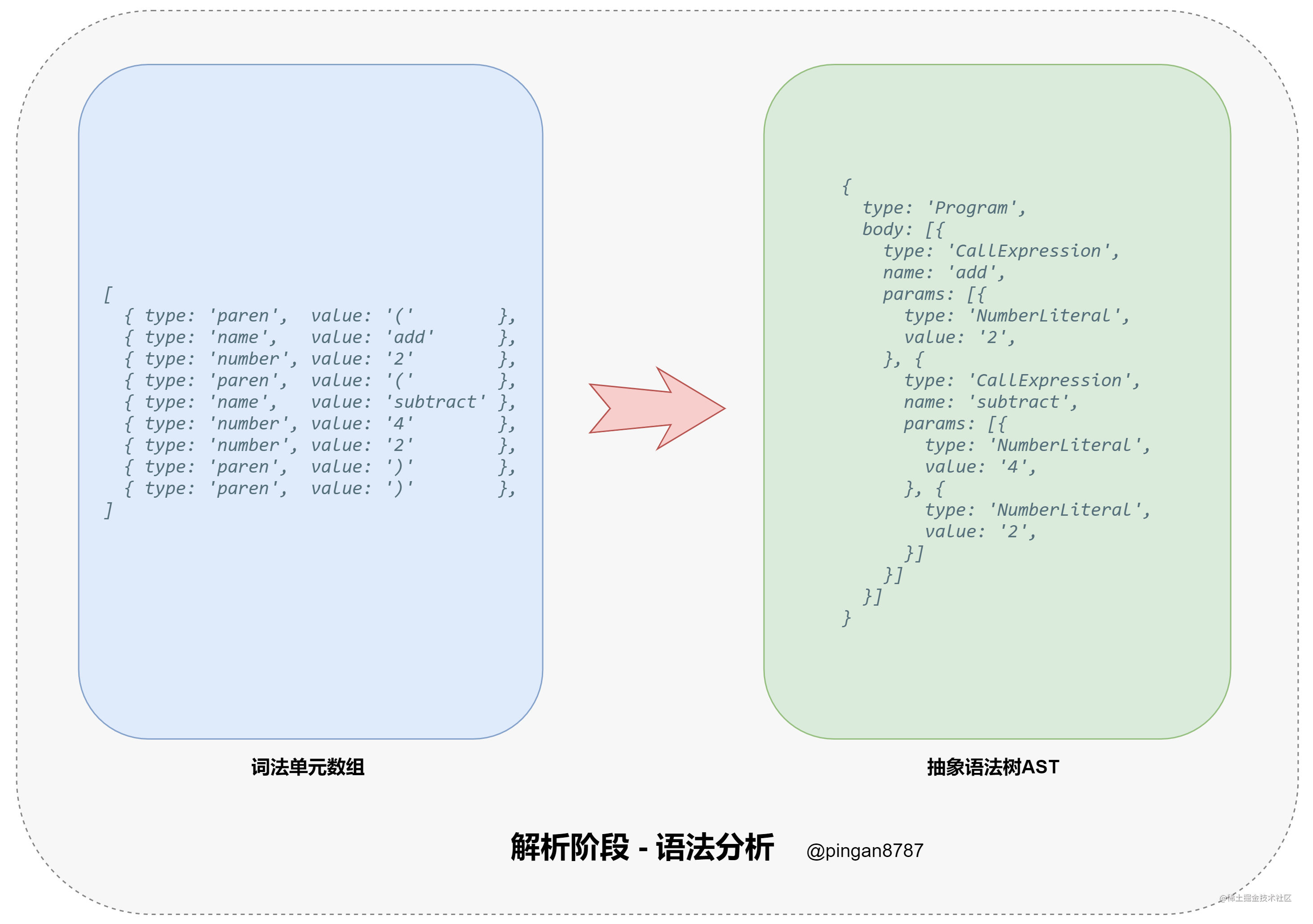1. 进入转换阶段（Transformation），将上一步生成的 AST 对象 导入转换器（Transformer），通过转换器中的遍历器（Traverser），将代码转换为我们所需的新的 AST 对象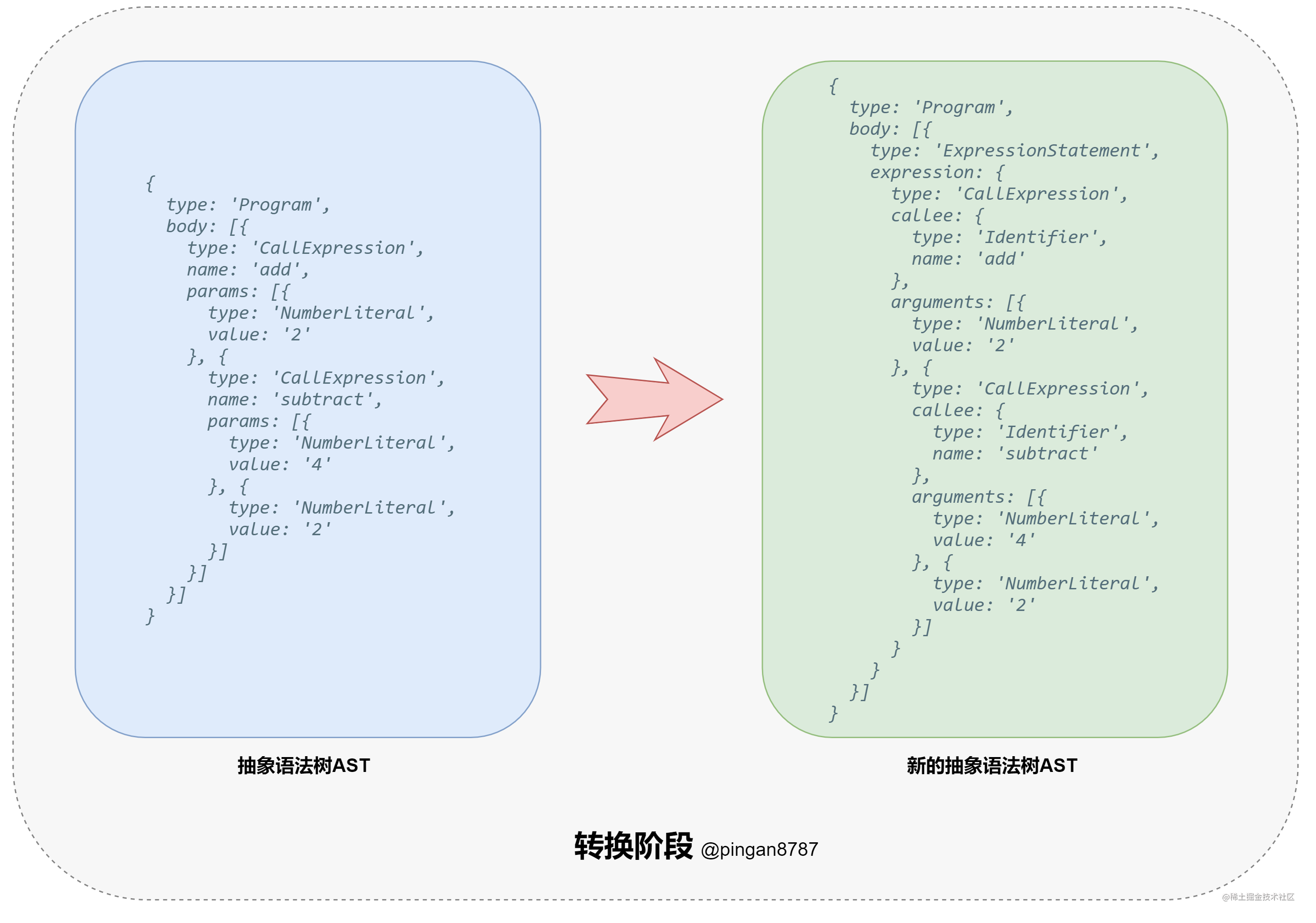1. 进入代码生成阶段（Code Generation），将上一步返回的新 AST 对象通过代码生成器（CodeGenerator），转换成 JavaScript Code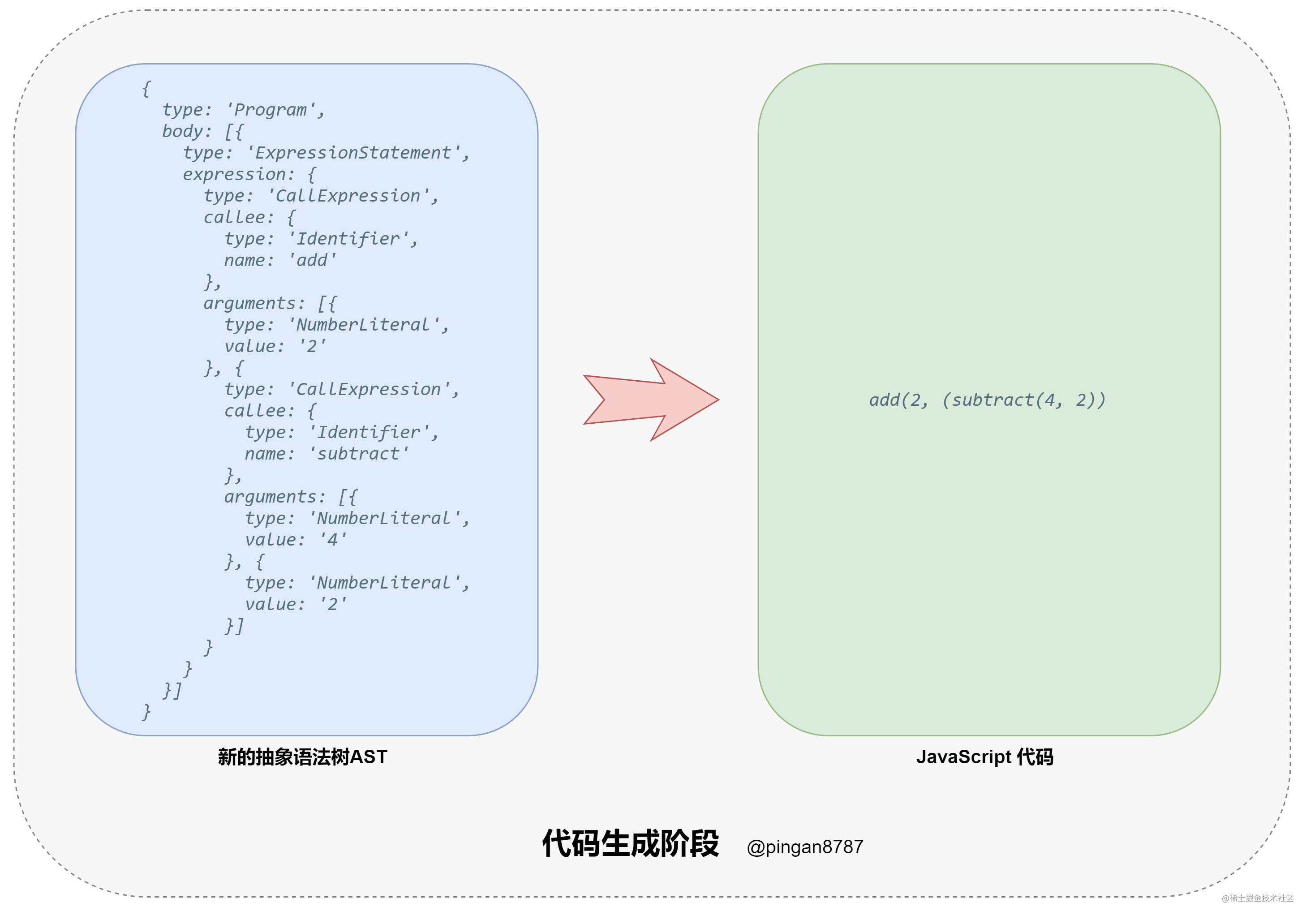1. 代码编译结束，返回 JavaScript Code## 3.2 入口方法

``````// 编译器入口方法 参数：原始代码字符串 input
function compiler(input) {
let tokens = tokenizer(input);
let ast    = parser(tokens);
let newAst = transformer(ast);
let output = codeGenerator(newAst);
return output;
}

## 3.3 解析阶段

``````// 词法分析器 参数：原始代码字符串 input
function tokenizer(input) {};

// 语法分析器 参数：词法单元数组tokens
function parser(tokens) {};

### 词法分析器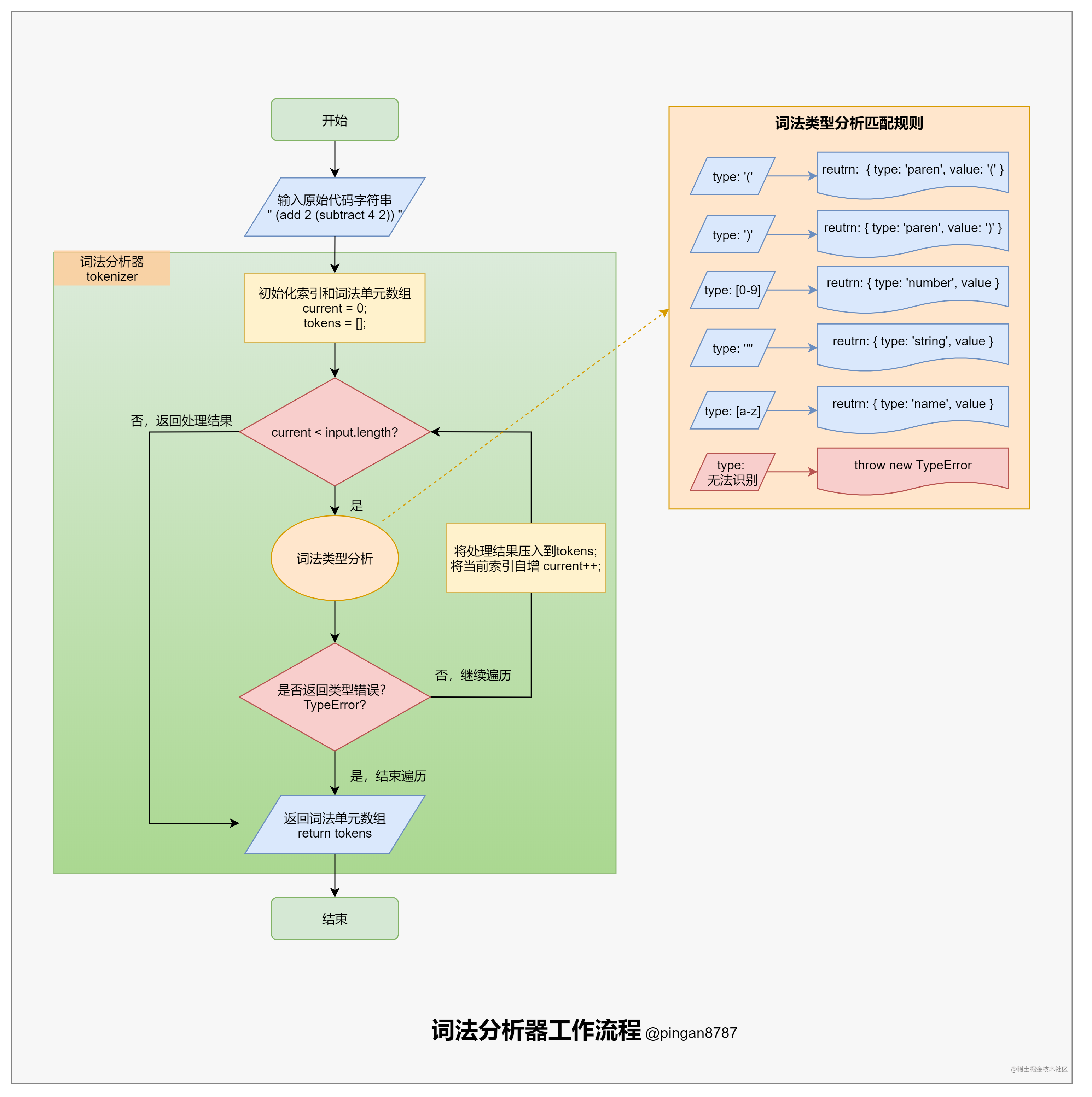``````// 词法分析器 参数：原始代码字符串 input
function tokenizer(input) {
let current = 0;  // 当前解析的字符索引，作为游标
let tokens = [];  // 初始化词法单元数组
// 循环遍历原始代码字符串，读取词法单元数组
while (current < input.length) {
let char = input[current];
// 匹配左括号，匹配成功则压入对象 {type: 'paren', value:'('}
if (char === '(') {
tokens.push({
type: 'paren',
value: '('
});
current++;
continue; // 自增current，完成本次循环，进入下一个循环
}
// 匹配右括号，匹配成功则压入对象 {type: 'paren', value:')'}
if (char === ')') {
tokens.push({
type: 'paren',
value: ')'
});
current++;
continue;
}

// 匹配空白字符，匹配成功则跳过
// 使用 \s 匹配，包括空格、制表符、换页符、换行符、垂直制表符等
let WHITESPACE = /\s/;
if (WHITESPACE.test(char)) {
current++;
continue;
}
// 匹配数字字符，使用 [0-9]：匹配
// 匹配成功则压入{type: 'number', value: value}
// 如 (add 123 456) 中 123 和 456 为两个数值词法单元
let NUMBERS = /[0-9]/;
if (NUMBERS.test(char)) {
let value = '';
// 匹配连续数字，作为数值
while (NUMBERS.test(char)) {
value += char;
char = input[++current];
}
tokens.push({ type: 'number', value });
continue;
}
// 匹配形双引号包围的字符串
// 匹配成功则压入 { type: 'string', value: value }
// 如 (concat "foo" "bar") 中 "foo" 和 "bar" 为两个字符串词法单元
if (char === '"') {
let value = '';
char = input[++current]; // 跳过左双引号
// 获取两个双引号之间所有字符
while (char !== '"') {
value += char;
char = input[++current];
}
char = input[++current];// 跳过右双引号
tokens.push({ type: 'string', value });
continue;
}
// 匹配函数名，要求只含大小写字母，使用 [a-z] 匹配 i 模式
// 匹配成功则压入 { type: 'name', value: value }
let LETTERS = /[a-z]/i;
if (LETTERS.test(char)) {
let value = '';
// 获取连续字符
while (LETTERS.test(char)) {
value += char;
char = input[++current];
}
tokens.push({ type: 'name', value });
continue;
}
// 当遇到无法识别的字符，抛出错误提示，并退出
throw new TypeError('I dont know what this character is: ' + char);
}
// 词法分析器的最后返回词法单元数组
}

### 语法分析器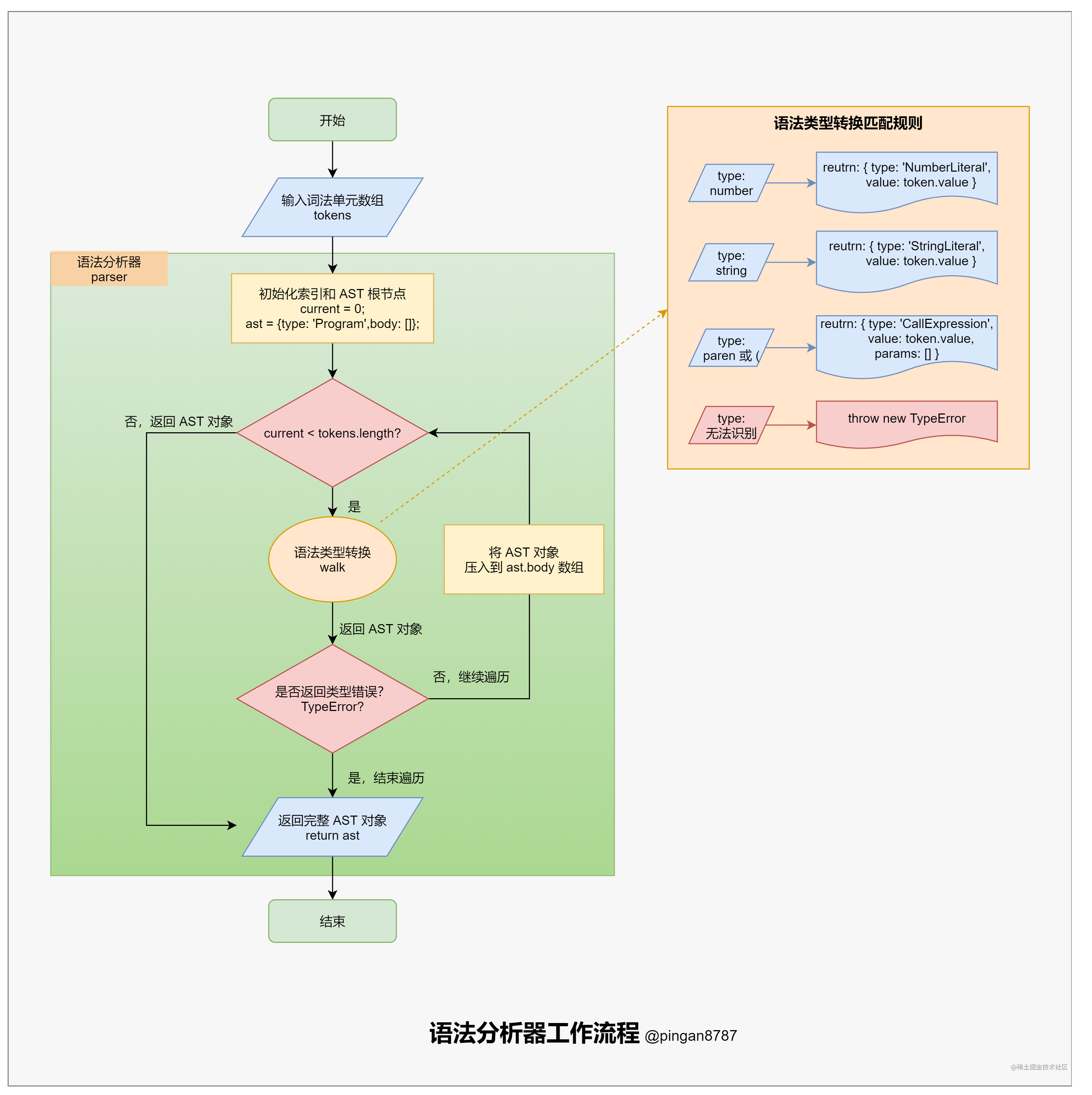``````// 语法分析器 参数：词法单元数组tokens
function parser(tokens) {
let current = 0; // 设置当前解析的词法单元的索引，作为游标
// 递归遍历（因为函数调用允许嵌套），将词法单元转成 LISP 的 AST 节点
function walk() {
// 获取当前索引下的词法单元 token
let token = tokens[current];

// 数值类型词法单元
if (token.type === 'number') {
current++; // 自增当前 current 值
// 生成一个 AST节点 'NumberLiteral'，表示数值字面量
return {
type: 'NumberLiteral',
value: token.value,
};
}

// 字符串类型词法单元
if (token.type === 'string') {
current++;
// 生成一个 AST节点 'StringLiteral'，表示字符串字面量
return {
type: 'StringLiteral',
value: token.value,
};
}

// 函数类型词法单元
if (token.type === 'paren' && token.value === '(') {
// 跳过左括号，获取下一个词法单元作为函数名
token = tokens[++current];

let node = {
type: 'CallExpression',
name: token.value,
params: []
};

// 再次自增 current 变量，获取参数词法单元
token = tokens[++current];

// 遍历每个词法单元，获取函数参数，直到出现右括号"）"
while ((token.type !== 'paren') || (token.type === 'paren' && token.value !== ')')) {
node.params.push(walk());
token = tokens[current];
}

current++; // 跳过右括号
return node;
}
// 无法识别的字符，抛出错误提示
throw new TypeError(token.type);
}

// 初始化 AST 根节点
let ast = {
type: 'Program',
body: [],
};

// 循环填充 ast.body
while (current < tokens.length) {
ast.body.push(walk());
}

// 最后返回ast
return ast;
}

## 3.4 转换阶段

``````// 遍历器 参数：ast 和 visitor
function traverser(ast, visitor) {
// 定义方法 traverseArray
// 用于遍历 AST节点数组，对数组中每个元素调用 traverseNode 方法。
function traverseArray(array, parent) {
array.forEach(child => {
traverseNode(child, parent);
});
}

// 定义方法 traverseNode
// 用于处理每个 AST 节点，接受一个 node 和它的父节点 parent 作为参数
function traverseNode(node, parent) {
// 获取 visitor 上对应方法的对象
let methods = visitor[node.type];
// 获取 visitor 的 enter 方法，处理操作当前 node
if (methods && methods.enter) {
methods.enter(node, parent);
}

switch (node.type) {
// 根节点
case 'Program':
traverseArray(node.body, node);
break;
// 函数调用
case 'CallExpression':
traverseArray(node.params, node);
break;
// 数值和字符串，忽略
case 'NumberLiteral':
case 'StringLiteral':
break;

// 当遇到无法识别的字符，抛出错误提示，并退出
default:
throw new TypeError(node.type);
}
if (methods && methods.exit) {
methods.exit(node, parent);
}
}
// 首次执行，开始遍历
traverseNode(ast, null);
}

``````// 转化器，参数：ast
function transformer(ast) {
// 创建 newAST，与之前 AST 类似，Program：作为新 AST 的根节点
let newAst = {
type: 'Program',
body: [],
};

// 通过 _context 维护新旧 AST，注意 _context 是一个引用，从旧的 AST 到新的 AST。
ast._context = newAst.body;

// 通过遍历器遍历 处理旧的 AST
traverser(ast, {
// 数值，直接原样插入新AST，类型名称 NumberLiteral
NumberLiteral: {
enter(node, parent) {
parent._context.push({
type: 'NumberLiteral',
value: node.value,
});
},
},
// 字符串，直接原样插入新AST，类型名称 StringLiteral
StringLiteral: {
enter(node, parent) {
parent._context.push({
type: 'StringLiteral',
value: node.value,
});
},
},
// 函数调用
CallExpression: {
enter(node, parent) {
// 创建不同的AST节点
let expression = {
type: 'CallExpression',
callee: {
type: 'Identifier',
name: node.name,
},
arguments: [],
};

// 函数调用有子类，建立节点对应关系，供子节点使用
node._context = expression.arguments;

// 顶层函数调用算是语句，包装成特殊的AST节点
if (parent.type !== 'CallExpression') {

expression = {
type: 'ExpressionStatement',
expression: expression,
};
}
parent._context.push(expression);
},
}
});
return newAst;
}

## 3.5 代码生成

``````// 代码生成器 参数：新 AST 对象
function codeGenerator(node) {

switch (node.type) {
// 遍历 body 属性中的节点，且递归调用 codeGenerator，按行输出结果
case 'Program':
return node.body.map(codeGenerator)
.join('\n');

// 表达式，处理表达式内容，并用分号结尾
case 'ExpressionStatement':
return (
codeGenerator(node.expression) +
';'
);

// 函数调用，添加左右括号，参数用逗号隔开
case 'CallExpression':
return (
codeGenerator(node.callee) +
'(' +
node.arguments.map(codeGenerator)
.join(', ') +
')'
);

// 标识符，返回其 name
case 'Identifier':
return node.name;
// 数值，返回其 value
case 'NumberLiteral':
return node.value;

// 字符串，用双引号包裹再输出
case 'StringLiteral':
return '"' + node.value + '"';

// 当遇到无法识别的字符，抛出错误提示，并退出
default:
throw new TypeError(node.type);
}
}

## 3.6 编译器测试

``````const add = (a, b) => a + b;
const subtract = (a, b) => a - b;
const source = "(add 2 (subtract 4 2))";
const target = compiler(source); // "add(2, (subtract(4, 2));"

const result = eval(target); // Ok result is 4

## 3.7 工作流程小结

1、input => tokenizer => tokens
2、tokens => parser => ast
3、ast => transformer => newAst
4、newAst => generator => output

# 四、手写 Webpack 编译器## 4.1 Webpack 构建流程分析

1. 初始化参数

1. 开始编译

1. 确定入口

1. 编译模块

1. 完成模块编译并输出

1. 输出完成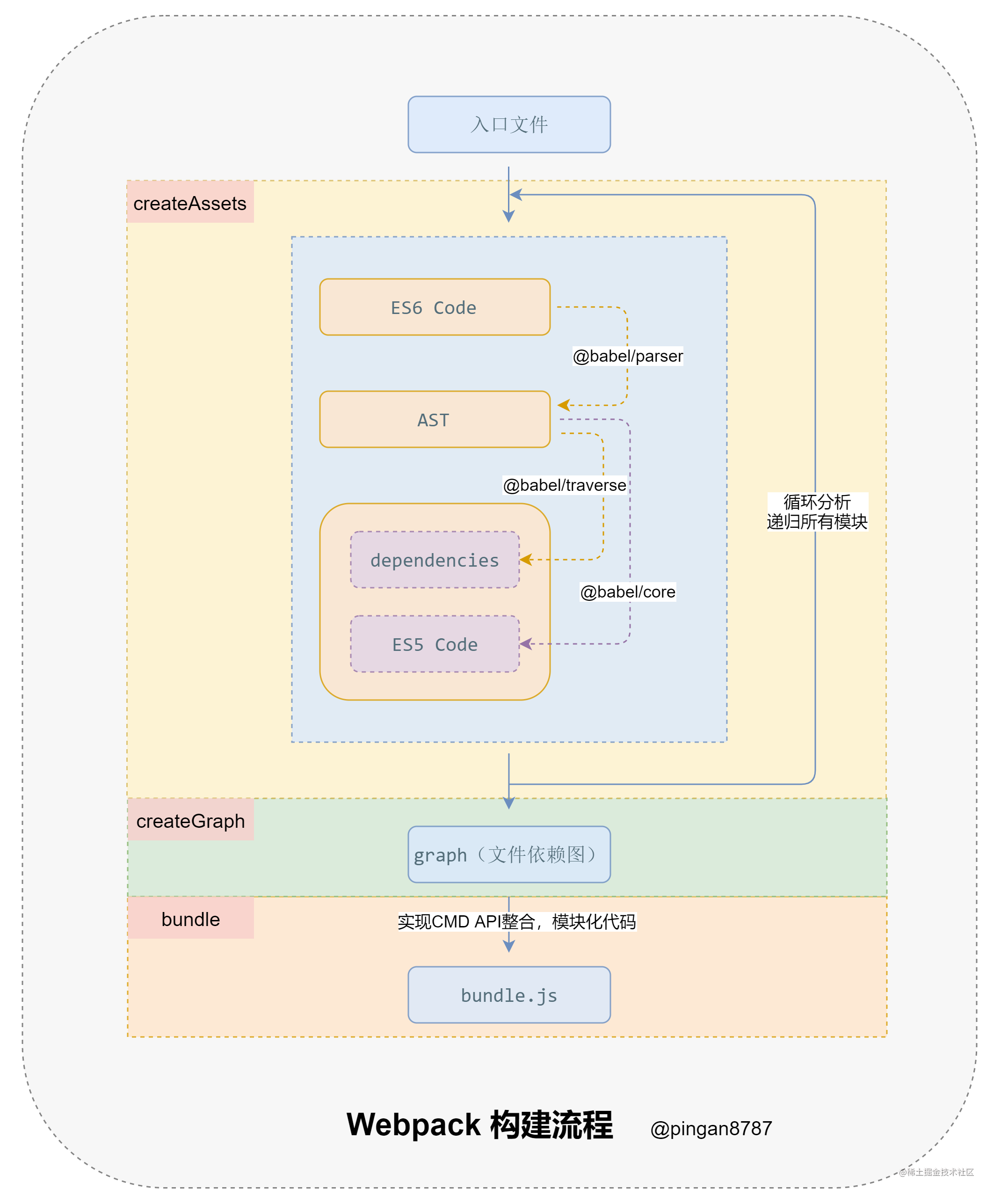## 4.2 代码实现

• `createAssets` : 收集和处理文件的代码；
• `createGraph` ：根据入口文件，返回所有文件依赖图；
• `bundle` : 根据依赖图整个代码并输出；

### 1. createAssets

``````function createAssets(filename){
const content = fs.readFileSync(filename, "utf-8"); // 根据文件名读取文件内容

// 将读取到的代码内容，转换为 AST
const ast = parser.parse(content, {
sourceType: "module" // 指定源码类型
})
const dependencies = []; // 用于收集文件依赖的路径

// 通过 traverse 提供的操作 AST 的方法，获取每个节点的依赖路径
traverse(ast, {
ImportDeclaration: ({node}) => {
dependencies.push(node.source.value);
}
});

// 通过 AST 将 ES6 代码转换成 ES5 代码
const { code } = babel.transformFromAstSync(ast, null, {
presets: ["@babel/preset-env"]
});

let id = moduleId++;
return {
id,
filename,
code,
dependencies
}
}

### 2. createGraph

``````function createGraph(entry) {
const mainAsset = createAssets(entry); // 获取入口文件下的内容
const queue = [mainAsset];
for(const asset of queue){
const dirname = path.dirname(asset.filename);
asset.mapping = {};
asset.dependencies.forEach(relativePath => {
const absolutePath = path.join(dirname, relativePath); // 转换文件路径为绝对路径
const child = createAssets(absolutePath);
asset.mapping[relativePath] = child.id;
queue.push(child); // 递归去遍历所有子节点的文件
})
}
return queue;
}

### 3. bunlde

``````function bundle(graph) {
let modules = "";
graph.forEach(item => {
modules += `
\${item.id}: [
function (require, module, exports){
\${item.code}
},
\${JSON.stringify(item.mapping)}
],
`
})
return `
(function(modules){
function require(id){
const [fn, mapping] = modules[id];
function localRequire(relativePath){
return require(mapping[relativePath]);
}

const module = {
exports: {}
}

fn(localRequire, module, module.exports);

return module.exports;
}
require(0);
})({\${modules}})
`
}

# 五、总结